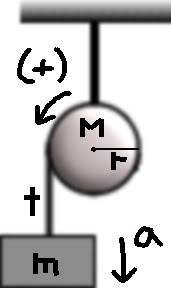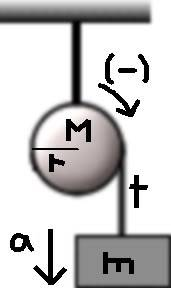# Torque and Tangential Acceleration

## Homework Statement

My ENTIRE AP physics class is stumped on the way to correctly write the formula to find acceleration for a falling block (mass of "m") attached by a string (tension of "t") to a fixed, rotating pulley (mass of "M", radius of "r"). Our teacher told us that for a block attached on the left side of a pulley (rotating counterclockwise) the tangential acceleration ("a") of the pulley should be positive (+) and for one on the right side (pulley rotating clockwise) a should be negative (-). We cannot come to a decisive conclusion as to what the final equation to find a for each side should be.

m = block mass
M = pulley mass
t = tension of string
a = tangential acceleration (acceleration of the block)
I = Inertia of pulley
A = angular acceleration of pulley
g = Gravity (assume g=10 if needed)

## Homework Equations

Positive "a" Formula Set:(+)Formula One: Pulley Rotating CCW

T = rt, T = IA , I = 1/2M(r^2), and A = a/r

therefore... rt = 1/2 M(r^2)(a/r)
(r^2)t = 1/2M(r^2)a
t = 1/2Ma

(+)Formula Two: Block Falling to the Left

t = sum of all forces
t = mg + ma

Negative "a" Formula Set:(-)Formula One: Pulley Rotating CW

T = rt, T = IA , I = 1/2M(r^2), and A = -a/r

therefore... rt = 1/2 M(r^2)(-a/r)
(r^2)t = 1/2M(r^2)(-a)
t = -(1/2Ma)

(-)Formula Two: Block Falling to the Right

t = sum of all forces
t = mg + [m(-a)]
or
t = mg - ma
(This part is where we some of the confusion begins. We assume t = mg "-" ma since the block is falling to the right and "a" should be negative)

## The Attempt at a Solution

Positive, CCW, Left

t = mg + ma
and
t = 1/2Ma
so
1/2Ma = mg + ma
both sides x 2
Ma = 2mg + 2ma
both sides -2ma
Ma - 2ma = 2mg
factor out a
a(M - 2m) = 2mg
both sides / (M-2m)
a = 2mg / (M - 2m)

Negative, CW, Right

t= mg - ma
and
t = -(1/2Ma)
so
-(1/2Ma) = mg - ma
both sides x-(2)
Ma = -2mg + 2ma
both sides -2ma
Ma - 2ma = -2mg
actor out a
a(M - 2m) = -2mg
both sides / (M - 2m)
a = -2mg / (M - 2m)

I've worked these out to the best of my ability and I believe that they are correct. Can someone please either confirm this or correct me ASAP?
Thanks!

AlephZero